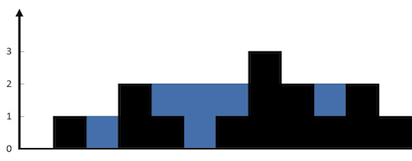#### The Rainwater Trapping Problem

At Viking Code School we’ve been challenging each other with algorithm problems. This week I decided to go with the rainwater trapping problem.

Given an array of positive integers representing an elevation map, imagine that rain water could be “trapped” by that elevation map. Write an algorithm to determine how much rainwater could be trapped.

Rainwater is assumed to “leak” out the side of the graph, and the size of “columns” and “rows” is assumed to be 1 to simplify calculations. To make this clearer, here’s a visualization for [0,1,0,2,1,0,1,3,2,1,2,1], which traps 6 units of water:Take some time to figure out out for yourself, if you are so inclined, before moving on to a couple of solutions below.

#### Two solutions

An approach that might seem to make sense at first is to replicate the visualization, but that requires turning a nice simple 1-dimensional array into a bloated 2-dimensional one. Then you have an n^2 problem, which we want to avoid!

One thing that does seem to be necessary is to iterate from both ends, and keep track of local and global maxima. This prevents missing a case like the one between two twos three spots from the end. Here are two solutions which follow this approach (see the Github Gist below).

The first is one I found online, and is pretty typical of ones you’ll see. Basically, in this version you have to loop through the array a bunch of times.

1. Build up arrays of the iterative maximum (maximum “so far” as you go through the array) going in one direction and then the other.
2. Then you go through again, building a new array of the smaller number from each of those arrays.
3. Subtract the original array from that one.
4. Return a the sum of the result.

Each of these steps requires a separate loop! Whew… getting tired just looking at it. Below that you can see my solution, which as far as I an tell works just as well and is much more efficient. Same O(n) but with a better constant and a best-case of (log n).

The second solution does basically the same thing in a single loop and a few extra variables (as opposed to 4 loops and a few extra arrays). Basically:

1. Start from both ends, keeping track of left and right indices.
2. Keep track of the max encountered by the left pointer, the max encountered by the right pointer, and the global max.
3. Keep track of the water which can be captured by each space, “dumping” it into the total at logical points (i.e. when encountering a new max).
4. Iterate pointers only when not at a global max (with some tie-breaker logic). This prevents pointers from having to look at more than one “well” of trapped water at once. Break when the pointers meet.

So the worst-case time complexity in this case is the same as the other approach, but with a much better best case. The best case would be the pointers meeting near the middle of the array, giving a complexity of (log n).

If you can think of a better approach, please post it in the comments!